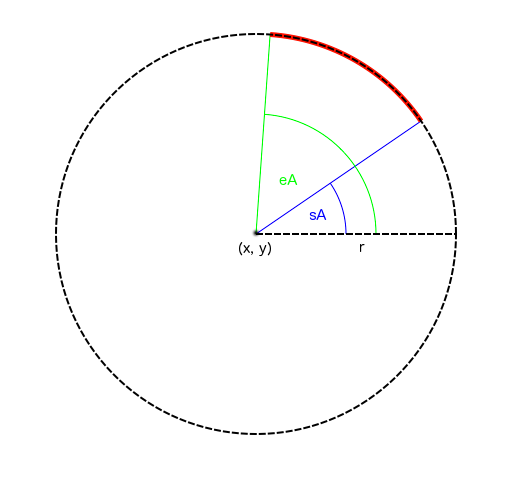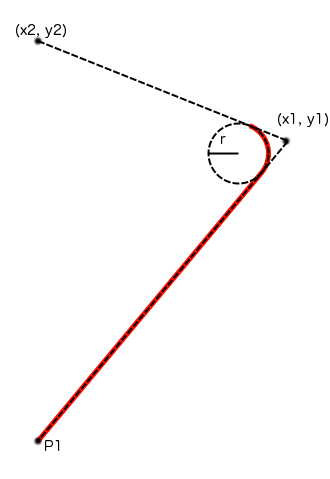Apple's CoreGraphics library defines two functions for describing an arc.

• CGPathAddArc adds an arc based on a center point, radius, and pair of angles.
• CGPathAddArcToPoint adds an arc based on a radius and a pair of tangent lines.

The details are explained in the CGPath API reference. Why two functions? Simple convenience? Is one more efficient than the other? Is one defined in terms of the other?

`CGContextAddArc` does this:where the red line is what will be drawn, sA is `startAngle`, eA is the `endAngle`, r is `radius`, and x and y are `x` and `y`. If you have a previous point the function will line from this point to the start of the arc (unless you are careful this line won't be going in the same direction as the arc).

`CGContextAddArcToPoint` works like this:Where P1 is the current point of the path, the x1, x2, y1, y2 match the functions `x1`, `x2`, `y1`, `y2` and r is `radius`. The arc will start in the same direction as the line between the current point and `(x1, y1)` and end in the direction between `(x1, y1)` and `(x2, y2)`. it won't line to `(x2, y2)` It will stop at the end of the circle.

• Excellent! I bet these are not the only two. Where can I find the diagrams for the other APIs? Jun 1, 2015 at 4:03
• @CopperCash Sorry to disappoint you, but these are the only two that I know of, and I drew them in code (mostly, the labels and points were added with an image editor). Jun 1, 2015 at 4:58

The former gets you a portion of a circle (really, an approximation of one), while the latter exposes the fact that you're creating a Bézier path. Depending on what you're actually drawing, one or the other might be more convenient. You could really consider both of them conveniences for CGPathAddCurveToPoint.

• Do you mean to imply that CGPathAddArcToPoint can draw a curve that is not circular? I thought all arcs are portions of a circle. Sep 17, 2008 at 19:32
• A circle cannot be described exactly by a Bezier curve. Oct 26, 2008 at 6:35